Related Articles

# Class 8 NCERT Solutions – Chapter 4 Practical Geometry – Exercise 4.2

• Last Updated : 04 May, 2021

### Question 1: Construct the following quadrilaterals.

LI = 4 cm
IF = 3 cm
TL = 2.5 cm
LF = 4.5 cm
IT = 4 cm

Solution:

Steps of construction:

Step 1: Draw a line of 4 cm and label it as LI
Step 2: Taking I as the centre draw an arc of radius 3cm
Step 3: Taking L as the centre draw an arc of radius 4.5cm, so that both the arcs from I and L intersect each other and marks the intersection point as F. consider the fig below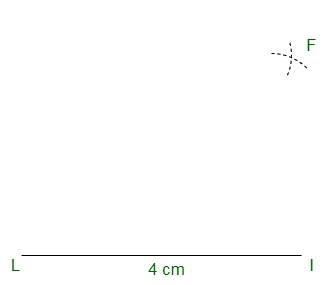Step 4: Join IF and LF
Step 5: Take L as centre draw an arc of radius 2.5 cm
Step 6: Take I as centre draw an arc of 4 cm to meet the previous arc and mark the intersection point as T.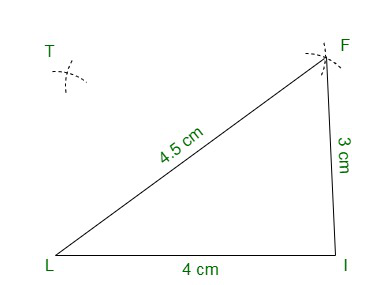Step 7: Join TF and TL
Step 8: Hence we have the required quadrilateral LIFT.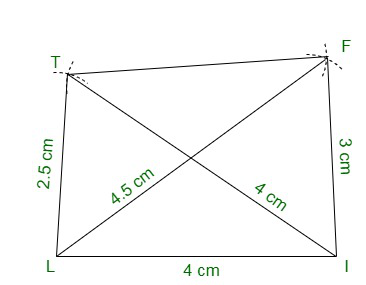OL = 7.5 cm
GL = 6 cm
GD = 6 cm
LD = 5 cm
OD = 10 cm

Solution:

Steps of construction:

Step 1: Draw a line OL of 7.5 cm
Step 2: Taking L as the centre draw an arc of 5 cm.
Step 3: Taking O as the centre draw an arc of 10 cm and let it intersect with the previous arc. Mark the intersection point as D as shown below: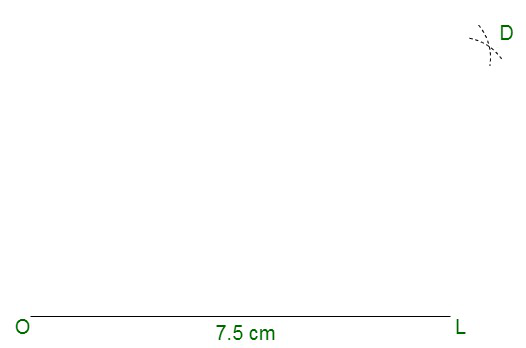Step 4: Join LD and OD.
Step 5: Take D as the centre and draw an arc of 6 cm.
Step 6: Now take L as the centre and drawn another arc of 6 cm that will intersect with the former arc at point G.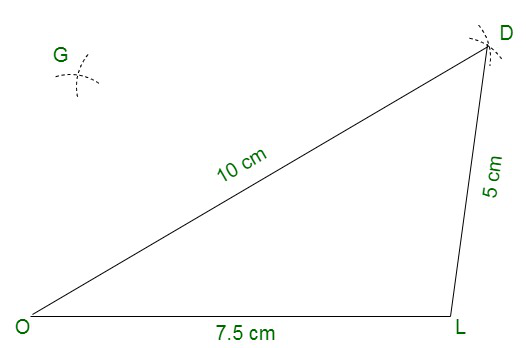Step 7: Join GL and GD.
Step 8: Hence we have the required quadrilateral GOLD.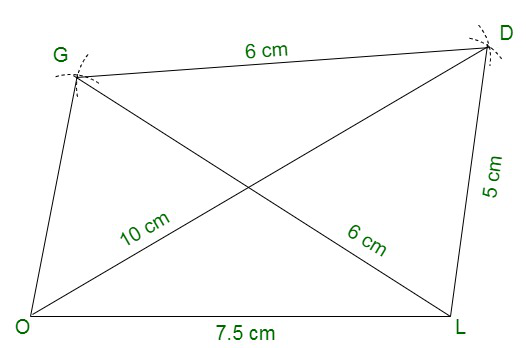(iii) Rhombus BEND
BN = 5.6 cm
DE = 6.5 cm

Solution:

Steps of construction:

Step 1: Draw a line BN of 5.6 cm
Step 2: Draw perpendicular bisector of BN and mark the bisector point as O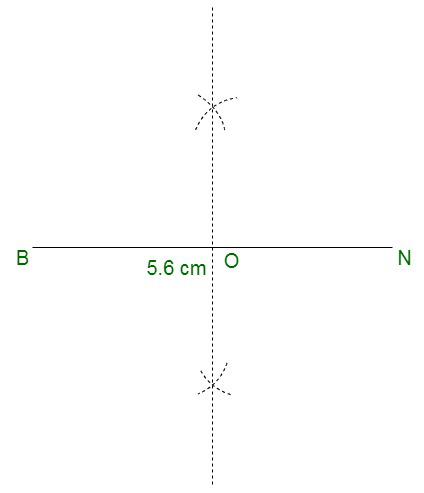Step 3:  Draw two arcs with centre O of radius  × DE =  × 6.5 cm = 3.25 cm and these arcs will meet the bisector at point D and E
2                2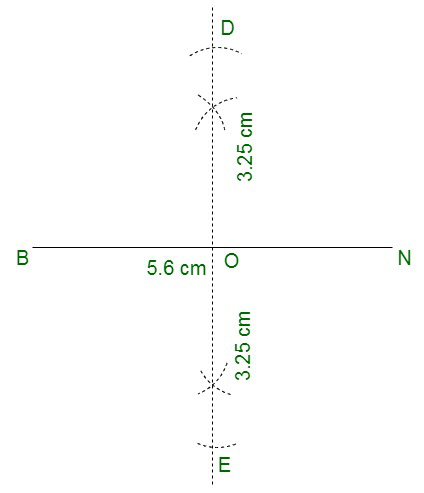Step 4: Join BE, EN, ND, and BD.
Step 5: Hence we have the required rhombus BEND.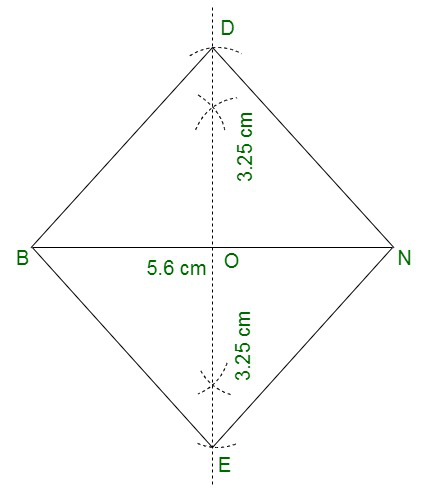Attention reader! All those who say programming isn’t for kids, just haven’t met the right mentors yet. Join the  Demo Class for First Step to Coding Coursespecifically designed for students of class 8 to 12.

The students will get to learn more about the world of programming in these free classes which will definitely help them in making a wise career choice in the future.

My Personal Notes arrow_drop_up